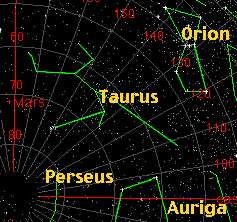Search IntMath
Close

450+ Math Lessons written by Math Professors and Teachers

5 Million+ Students Helped Each Year

1200+ Articles Written by Math Educators and Enthusiasts

Simplifying and Teaching Math for Over 23 Years

# Applications of Differentiation

by M. Bourne

## Introduction to Applications of Differentiation

In Isaac Newton's day, one of the biggest problems was poor navigation at sea.Before calculus was developed, the stars were vital for navigation.

Shipwrecks occured because the ship was not where the captain thought it should be. There was not a good enough understanding of how the Earth, stars and planets moved with respect to each other.

Calculus (differentiation and integration) was developed to improve this understanding.

Differentiation and integration can help us solve many types of real-world problems.

We use the derivative to determine the maximum and minimum values of particular functions (e.g. cost, strength, amount of material used in a building, profit, loss, etc.).

Derivatives are met in many engineering and science problems, especially when modelling the behaviour of moving objects.

Our discussion begins with some general applications which we can then apply to specific problems.

### Related Sections in "Interactive Mathematics"

Introduction to Calculus, where there is a brief history of calculus.

The Derivative, an introduction to differentiation, for those who have never heard of it.

Differentiation of Transcendental Functions, which shows how to find derivatives of sine, cosine, exponential and tangential functions.

Integration, which is actually the opposite of differentiation.

Differential Equations, which are a different type of integration problem, but still involve differentiation.

### In this Chapter

1. Tangents and Normals which are important in physics (eg forces on a car turning a corner)

2. Newton's Method - for those tricky equations that you cannot solve using algebra

3. Curvilinear Motion, which shows how to find velocity and acceleration of a body moving in a curve

4. Related Rates - where 2 variables are changing over time, and there is a relationship between the variables

5. Curve Sketching Using Differentiation, where we begin to learn how to model the behaviour of variables

6. More Curve Sketching Using Differentiation

7. Applied Maximum and Minimum Problems, which is a vital application of differentiation

8. Radius of Curvature, which shows how a curve is almost part of a circle in a local region

We begin with 1. Tangents and Normals »

## Problem SolverThis tool combines the power of mathematical computation engine that excels at solving mathematical formulas with the power of GPT large language models to parse and generate natural language. This creates math problem solver thats more accurate than ChatGPT, more flexible than a calculator, and faster answers than a human tutor. Learn More.